rates of change
derivatives

# How do I calculate rates? Calculating changes through time in the geosciences

## Introduction to ratesChange and time are two of the main themes in the geosciences. For example, geomorphologists study changes in landscape such as beach erosion, geochemists study chemical changes in rocks as they are weathered, climatologists study long-term changes in global and regional weather and climate. Because geologists are interested in how rapidly changes can occur, we also look at the time over which those changes took place. Combined, this creates a rate, the rapidity in which change occurs.

## What is a rate?

Any change with respect to time is called a rate.

This is represented mathematically by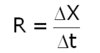Where R is the rate, ΔX is the change in whatever you are looking at (it could be temperature, pressure, distance, or anything else) and Δt is the change in time. In mathematics and many science fields, Δ means "change".
Some people use a formula that explicitly shows the subtraction to determine ΔX and Δt:
ΔX = X2-X1 and Δt = t2-t1
However many students can figure it out using without these additional formulas.

There are many rates in the geosciences - plate tectonic velocities (distance over time), crystal growth rates (change in crystal size over time), river and groundwater discharge (volume change over time), decay rates (change in number of parent isotopes over time), or any variable that is divided by time.Velocity (change in position divided by time) is the most common type of rate calculated in the geosciences and is commonly expressed in kilometers per million years, cm per year, km per hour, cm per second, etc. Most people have heard the expression "rate equals distance over time" (or the more accurate version "velocity equals distance over time"). This can be written in equation form as: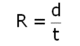or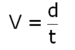where d = distance, t = time, R = rate and V = velocity. This is just a specific example of a rate because distance (d) is the change in position, ΔX.

## How do I calculate a rate?

Here are some steps for calculating a rate. Under each step you can find an example in which we determine a velocity of a glacier's retreat.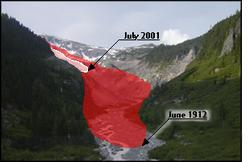In 1892, the terminus of Nisqually Glacier, on the southern side of Mt. Rainier in Washington, was located 40 meters above the current position of the Nisqually river bridge. In 1951, the terminus was located 790 m above the bridge. Calculate Nisqually Glacier's rate of retreat from the bridge (in m per year) between 1892 and 1951.
The steps to answer this question are:
1. Determine which changing variable is ΔX and which is Δt.
2. Calculate ΔX and Δt to determine the change in the variable(s).
3. Calculate the rate using ΔX and Δt.
4. Check to see what the units on your final number should be.

## Determining rates from a graph

Geoscientists often present data in a graph. Any graph that has time as the horizontal axis can be used to determine a rate. In these cases, the rate is the slope of the line on the graph (many of you will know this as "rise over run") or the change in the vertical axis variable divided by the change in time (on the horizontal axis).

For practice, let's take a look at the plot at the right (you can click on it to make it bigger). In 1950, population was approximately 2.5 billion people. In 2000, world population had grown to a little over 6 billion people. So, let's calculate the rate of population growth from 1950 to 2000.
Slope is the rise over the run; this is the same as our equation for a rate since the change in time on the horizontal axis and is thus the "run."
One slightly confusing thing is that our change in population, ΔX, is on the vertical axis (sometimes called the y-axis,) while Δt is on the horizontal axis (often called the x-axis.) To avoid confusion, use the rate equation above or "slope is rise over run."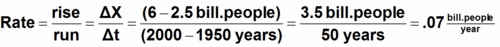So the rate of population growth between 1950 and 2000 is 0.07 billion people per year (that is 70 million people added to the planet every year on average!).

## Where are rates used in geology?

Rates are used in a range of topics in introductory geoscience courses:

• plate tectonics
• rocks (igneous, metamorphic and sedimentary)
• Earth history
• streams and groundwater
• glaciers
• mountain building and erosion
• climate change
• population growth
• and almost any other chapter in your textbook.
You will probably revisit the concept of rates many times - you can always come back to review how to calculate a rate!

## Next Steps

I think I understand how to calculate rates! Take me to the practice problems!

I still need more help! Continue below to get more help.

## I know the rate, this question is asking for something different!

Sometimes we have information about the rate but are asked to solve for another variable (time, distance, volume, etc.). The basic equation is still the same but you will need to rearrange it to solve for a different variable. The Math You Need, When You Need It has an entire module dedicated to rearranging equations (if you click that link, don't forget to come back and practice your newly learned steps!). The Rearranging Equations sample problems have several problems (right at the top of the page) that help you solve for distance and/or time if you know the velocity!

## Resources for rates and calculating velocity

Reaction rates in chemistry helps students calculate reaction rates for chemical reactions.

Calculating rates of change and slope for a graph describes and explains the process of finding the slope of a function, and thus the rate. This is from M. Casco Learning Center and has some nice mathematical applets.

by Dr. Eric M. Baer, Geology Program, Highline Community College and Dr. Jennifer M. Wenner, Geology Department, University of Wisconsin Oshkosh